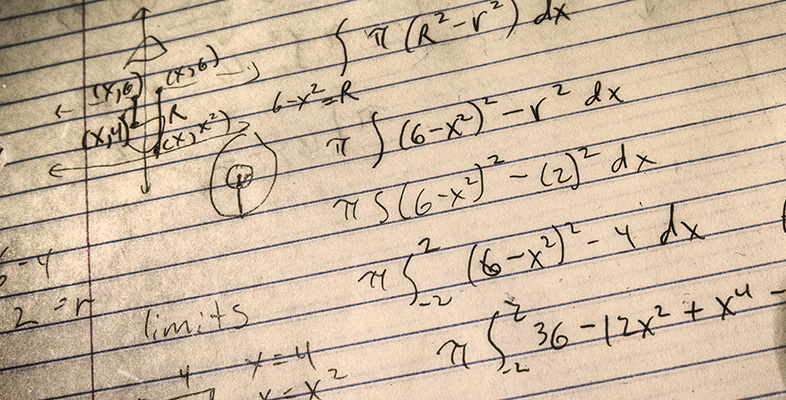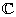An introduction to complex numbers

Start this free course now. Just create an account and sign in. Enrol and complete the course for a free statement of participation or digital badge if available.

Free course

# 1 An introduction to complex numbers

## 1.1 Workbook contents

The main teaching text of this course is provided in the workbook below. The answers to the exercises that you'll find throughout the workbook are given in the answer book. You can access it by clicking on the link under the workbook. Section 4.2 of the course requires you to listen to some audio files. You'll find these on the next page of this course.

Click on the link below to open the workbook (PDF, 4 MB).

Workbook [Tip: hold Ctrl and click a link to open it in a new tab. (Hide tip)]

Click on the link below to open the answer book (PDF, 4.2 MB).

• Introduction

• Study Guide

• 1 Introducing complex numbers

• 1.1 What is a complex number?

• 1.2 Arithmetic in• 1.3 Identities with complex numbers

• 2 The complex plane

• 2.1 Cartesian coordinates

• 2.2 Polar form

• 2.3 The geometric interpretation of multiplication and division

• 2.4 de Moivre's Theorem

• 3 Solving equations with complex numbers

• 3.1 Calculating nth roots

• 3.2 Solutions of polynomial equations

• 4 Sets of complex numbers

• 4.1 Inequalities

• 4.2 Sketching subsets of the complex plane (audio tape)

• 5 Proving inequalities

• 5.1 Rules for rearranging inequalities

• 5.2 The triangle inequality

M337_1